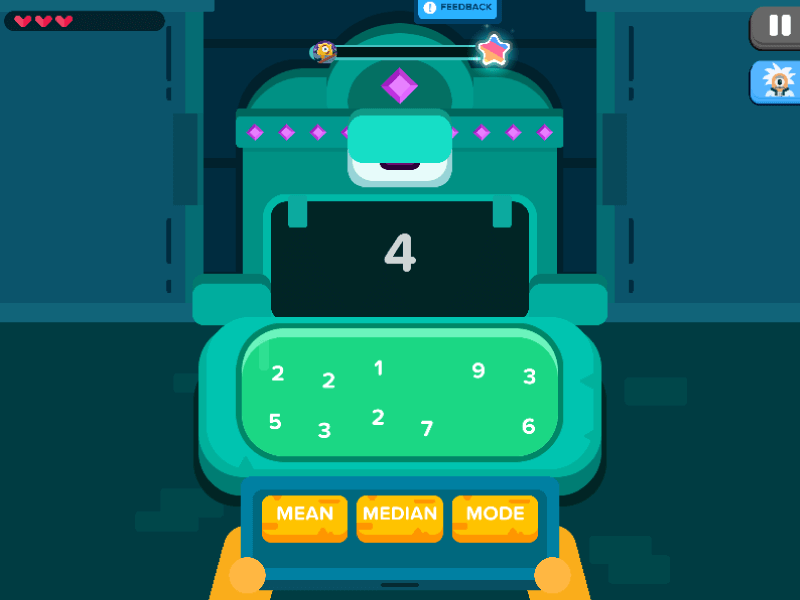## Statistic And Probability : Ancient Enigma### Ancient Enigma

The knowledge of statistic and probability is hidden inside the Zapzapmath Amazon Jungle. You have explored a multitude of its gorgeous scenic views and visits, but there is one you have yet to discover: the Ancient Enigma.

The Ancient Enigma is fast asleep. You approach it cautiously, and it awakens with a loud grunt. It looks at you expectedly, awaiting its meal. You look around and see three gold tablets lying on the floor. Each gold tablet has a word engraved on it: Mean, Median, and Mode. Which would the Ancient Enigma like to eat?

The stone may not speak a word, but it shows you a number to indicate which tablet it would like. To find the answer to this question, you need to study the small numbers shown on the head stone below its mouth. With the number provided, use your knowledge of statistic and probability to calculate the mean, median, and mode to see which matches the main number that the Ancient Enigma is asking for. This will be the correct tablet. To feed the stone, drag and drop the chosen tablet into its mouth.

If you get the answer right, you will be showered with gems. If you get the answer wrong, the Ancient Enigma will spit the tablet out, and be disgruntled by your error.

Ancient Enigma is an engaging game filled with cryptic mathematical problems to be solved. In this unit of inquiry, you will be studying the topic of statistic and probability especially how to define and calculate three different methods of calculations in the study of Mean, Median, and Mode.

The mean is the number that ties all the numbers in a line. To obtain the value of the mean, you add up all the numbers and divide the result by the total of numbers that you added up.

The median is the “middle” value in the list of numbers. To find the median, start by listing the numbers provided in numerical order from the smallest value to the largest. Find the middle value, and that is your median. If you have an odd number of integers, the median will be the number directly located in the middle of your number line. If you have an even amount of integers, you will need to add the two middle numbers up, and divide the answer by two. This will reveal your median.

The mode is the result that occurs the most. If no number in the list repeats itself, then there is no mode for that specific list. By mastering at least one of these three methods of statistics calculations, subsequent comprehension of the rest will follow suit. Now go forth and uncover the enigma with the power of statistic and probability.